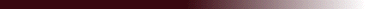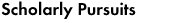## "Wavelet Transforms of the Navier-Stokes Equations and the Generalized Dimensions of Turbulence"

### The generalized dimensions D_q defined in the multifractal description of turbulence are related to the Navier-Stokes equations, and equations are presented for D_q and its evolution. In order to reach this result, the equations for incompressible flows are wavelet-transformed. When the analyzing wavelets belong in the Gaussian family, the pressure and momentum equations are transformed into first-order wave equations, for which the characteristics are obtained explicitly. Formal integration is carried out. As in Meneveau (1991), fractal statistics are then constructed from the local energy spectrum.

Appl. Sci. Res. 51, 109-113, 1993.

Jacques Lewalle, jlewalle@syr.edu